﻿ 溴化锂冷水机组在水下航行器中的性能研究
«上一篇文章快速检索 高级检索

 应用科技2020, Vol. 47Issue (4): 88-94  DOI: 10.11991/yykj.2019110220

### 引用本文XIA Junbao, LI Yi, LI Chunmei, et al. Performance research of lithium bromide chiller in underwater vehicle[J]. Applied Science and Technology, 2020, 47(4): 88-94. DOI: 10.11991/yykj.201911022.### 文章历史

Performance research of lithium bromide chiller in underwater vehicle
XIA Junbao, LI Yi, LI Chunmei, LI Weiwei, WU Zhisheng, SUN Guanyu, HAO Chengming, ZHAO Qianli, GAN Yiran
State Key Laboratory of Nuclear Reactor System Design Technology, Nuclear Power Institute of China, Chengdu 610213, China
Abstract: The performance research of lithium bromide chiller in underwater vehicle is studied in this paper. Firstly, the heat-exchange equipment of lithium bromide chiller was modeled, and the lsqnonlin minimization solution of constraint was carried out by Matlab programming, and the correctness of model was verified by the experiment. Secondly, the model was used to analyze the performance of the lithium bromide chiller. Finally, the energy consumption of the chiller of a certain underwater vehicle was quantitatively calculated. The results show that: the unit COP is 1.07~1.38 in the field test, and its maximum relative error is 2.94% compared with model calculated COP, meeting the accuracy requirements; heat source temperature, the cooling water inlet temperature and the chilled water inlet temperature have a greater impact on the unit COP; the unit COP of the underwater vehicle is 1.29, the dead steam consumption is 0.380 kg/s, and the unit cooling steam consumption is 1.366 kg/kW·h, which only accounts for 0.6% of the secondary circuit steam flow. There is basically no impact on the endurance capacity of the underwater vehicle.
Keywords: underwater vehicle    lithium bromide chiller    coefficient of performance (COP)    lsqnonlin minimization    endurance capacity    steam consumption    performance research    orthogonal optimization

1 溴化锂吸收式冷水机组模型建立

1.1 溴化锂吸收式冷水机组流程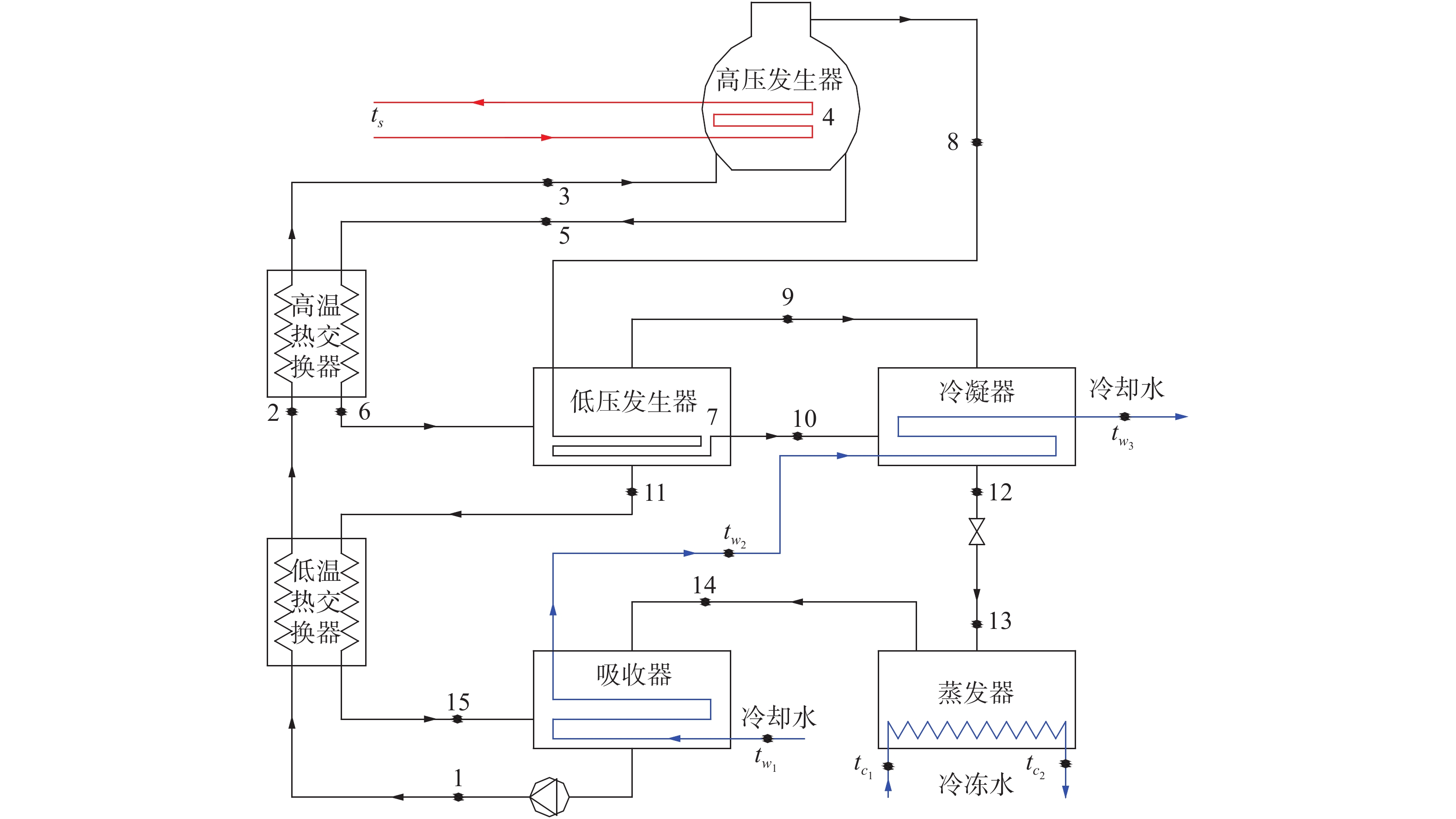Download: 图 1 溴化锂吸收式冷水机组流程
1.2 溴化锂吸收式冷水机组模型假设

1) 系统处于稳态；

2) 低压发生器压力等于冷凝压力；

3) 除热交换器外，所有换热设备出口均为饱和状态；

4) 系统不向环境散热；

5) 系统无电耗。

1.3 溴化锂吸收式冷水机组控制方程

1) 蒸发器

 ${Q_{0,1}} = D({h_{14}} - {h_{13}})$
 ${Q_{0,2}} = {C_p}{G_0}({t_{{c_1}}} - {t_{{c_2}}})$
 ${Q_{0,3}} = {K_0}{F_0}\dfrac{{{t_{{c_1}}} - {t_{{c_2}}}}}{{\ln \dfrac{{{t_{{c_1}}} - {t_0}}}{{{t_{{c_2}}} - {t_0}}}}}$

 $\dfrac{1}{{{K_i}}} = \dfrac{1}{{{\alpha _{i,{\rm{out}}}}}} + \dfrac{1}{{{\alpha _{i,{\rm{in}}}}}}\left(\dfrac{{{d_{i,{\rm{out}}}}}}{{{d_{i,{\rm{in}}}}}}\right) + \dfrac{{{d_{i,{\rm{out}}}}}}{{2{\lambda _i}}}\ln\dfrac{{{d_{i,{\rm{out}}}}}}{{{d_{i,{\rm{in}}}}}}(i = 0)$

2) 吸收器

 ${Q_{A,1}} = D\left((a - 1){h_{15}} + {h_{14}} - a{h_1}\right)$
 ${Q_{A,2}} = {C_p}{G_w}({t_{{w_2}}} - {t_{{w_1}}})$
 $a = \frac{{{x_h}}}{{{x_h} - {x_l}}}$
 ${G_1}{x_l} = ({G_1} - D){x_h}$
 ${Q_{A,3}} = {K_A}{F_A}\dfrac{{{t_{{w_2}}} - {t_{{w_1}}}}}{{\ln \dfrac{{{t_A} - {t_{{w_2}}}}}{{{t_A} - {t_{{w_1}}}}}}}$

3) 冷凝器

 ${Q_{k,1}} = D((1 - y){h_9} + y{h_{10}} - {h_{12}})$
 ${Q_{k,2}} = {C_p}{G_w}({t_{{w_3}}} - {t_{{w_2}}})$
 ${Q_{k,3}} = {K_k}{F_k}\dfrac{{{t_{{w_3}}} - {t_{{w_2}}}}}{{\ln \dfrac{{{t_k} - {t_{{w_2}}}}}{{{t_k} - {t_{{w_3}}}}}}}$

4) 低温热交换器

 ${Q_{{\rm{lex}},1}} = aD({h_2} - {h_1})$
 ${Q_{{\rm{lex}},2}} = (a - 1)D({h_{11}} - {h_{15}})$
 ${Q_{{\rm{lex}},3}} = {K_{{\rm{lex}}}}{F_{{\rm{lex}}}}\dfrac{{({t_{11}} - {t_2}) - ({t_{15}} - {t_1})}}{{\ln \dfrac{{{t_{11}} - {t_2}}}{{{t_{15}} - {t_1}}}}}$

5) 高温热交换器

 ${Q_{{\rm{hex,1}}}} = aD({h_3} - {h_2})$
 ${Q_{{\rm{hex}},2}} = (a - y)D({h_5} - {h_6})$
 ${Q_{{\rm{hex}},3}} = {K_{{\rm{hex}}}}{F_{{\rm{hex}}}}\dfrac{{({t_5} - {t_3}) - ({t_6} - {t_2})}}{{\ln \dfrac{{{t_5} - {t_3}}}{{{t_6} - {t_2}}}}}$

6) 低压发生器

 ${Q_{\lg ,1}} = D((a - 1){h_{11}} + (1 - y){h_9} - (a - y){h_6})$
 ${Q_{\lg ,2}} = yD({h_8} - {h_{10}})$
 $(a - y){x_m} = (a - 1){x_h}$
 ${Q_{\lg ,3}} = {K_{\lg }}{F_{\lg }}\dfrac{{{t_{11}} - {t_7}}}{{\ln \dfrac{{{t_{10}} - {t_7}}}{{{t_{10}} - {t_{11}}}}}}$

7) 高压发生器

 ${Q_{{\rm{hg}},1}} = D((a - y){h_5} + y{h_8} - a{h_3})$
 ${Q_{{\rm{hg}},2}} = \gamma {G_v}$
 $a{x_l} = (a - y){x_m}$
 ${Q_{{\rm{hg}},3}} = {K_{{\rm{hg}}}}{F_{{\rm{hg}}}}\dfrac{{{t_5} - {t_4}}}{{\ln \dfrac{{{t_s} - {t_4}}}{{{t_s} - {t_5}}}}}$
1.4 溴化锂吸收式冷水机组模型求解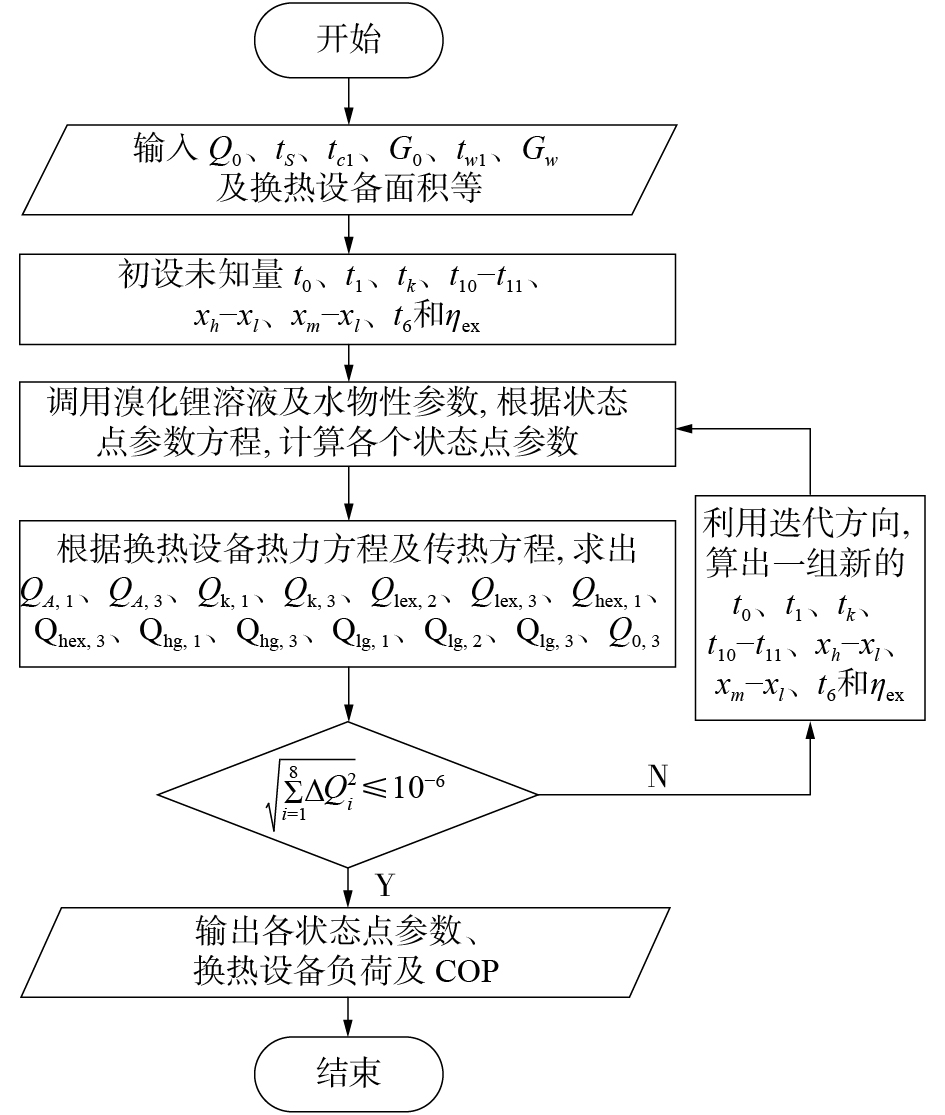Download: 图 2 溴化锂吸收式冷水机组数学模型流程
2 实测验证及性能分析

2.1 数学模型验证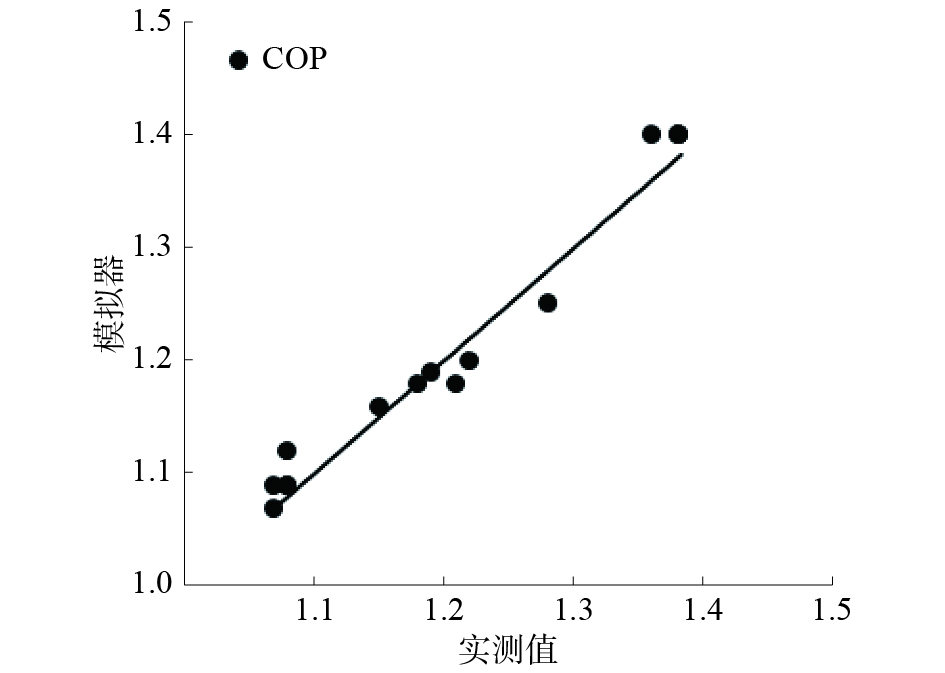Download: 图 3 溴化锂吸收式冷水机组模拟与实测结果对比
2.2 性能分析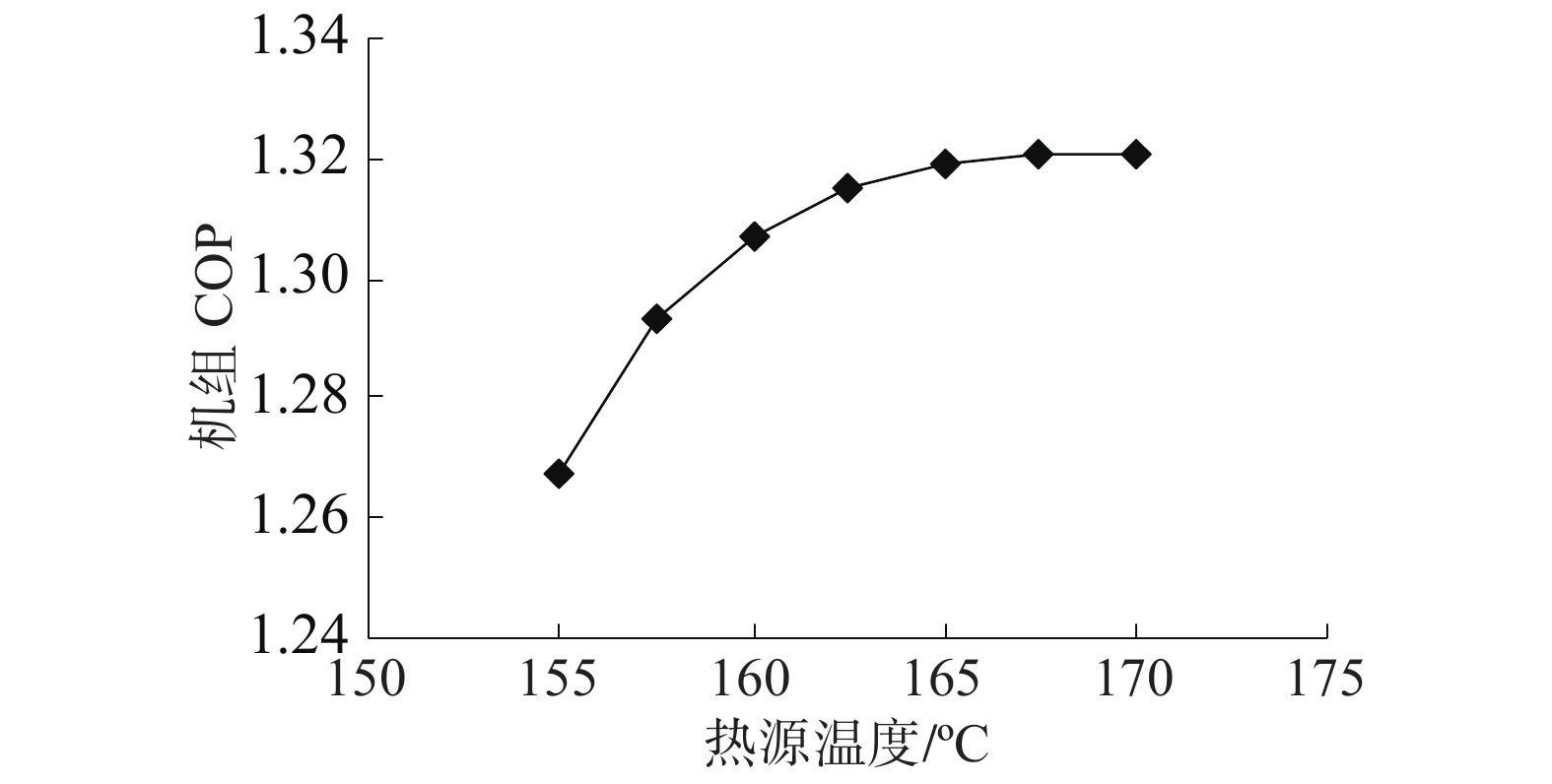Download: 图 4 热源温度对机组COP的影响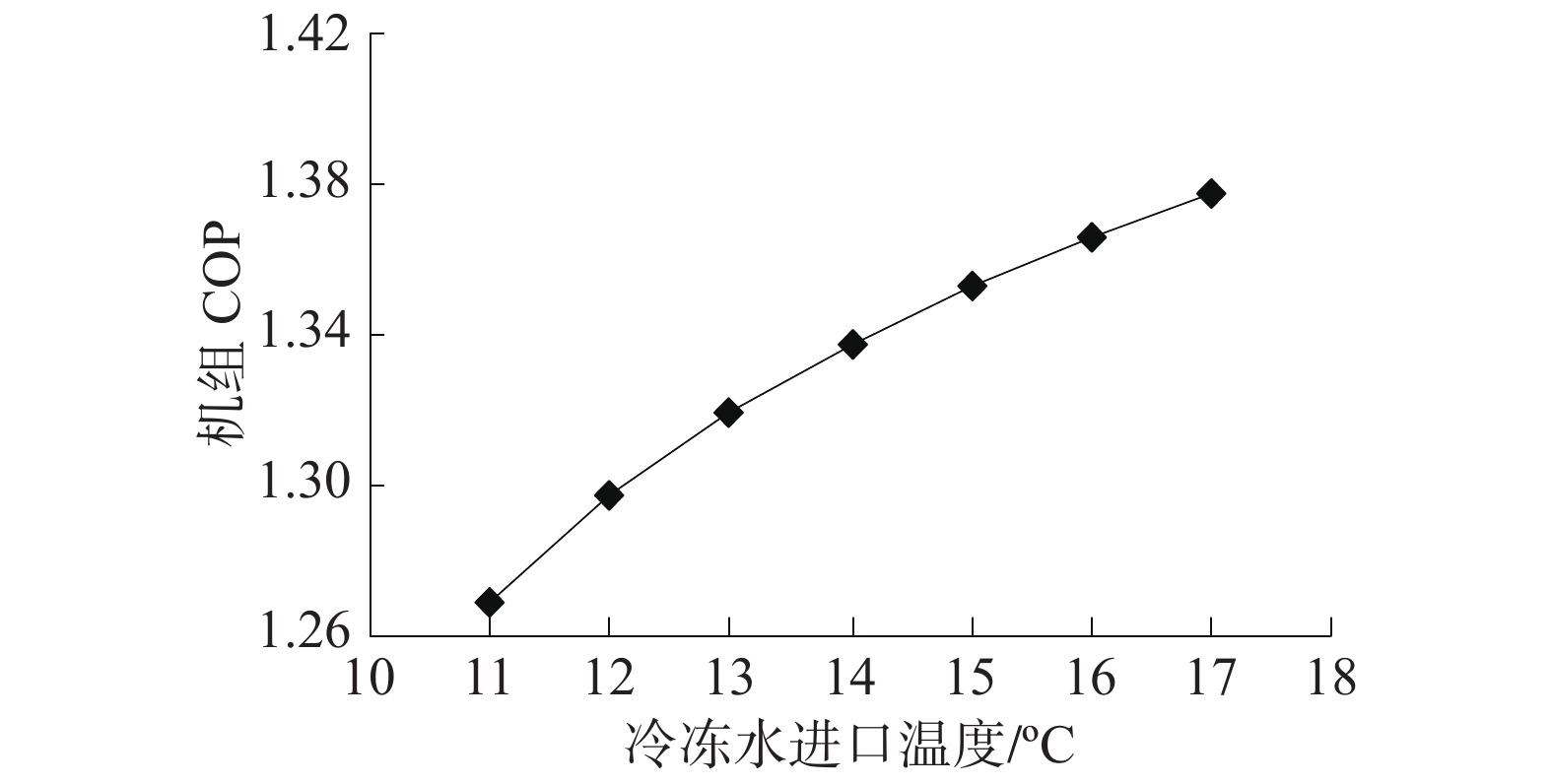Download: 图 5 冷冻水进口温度对机组COP的影响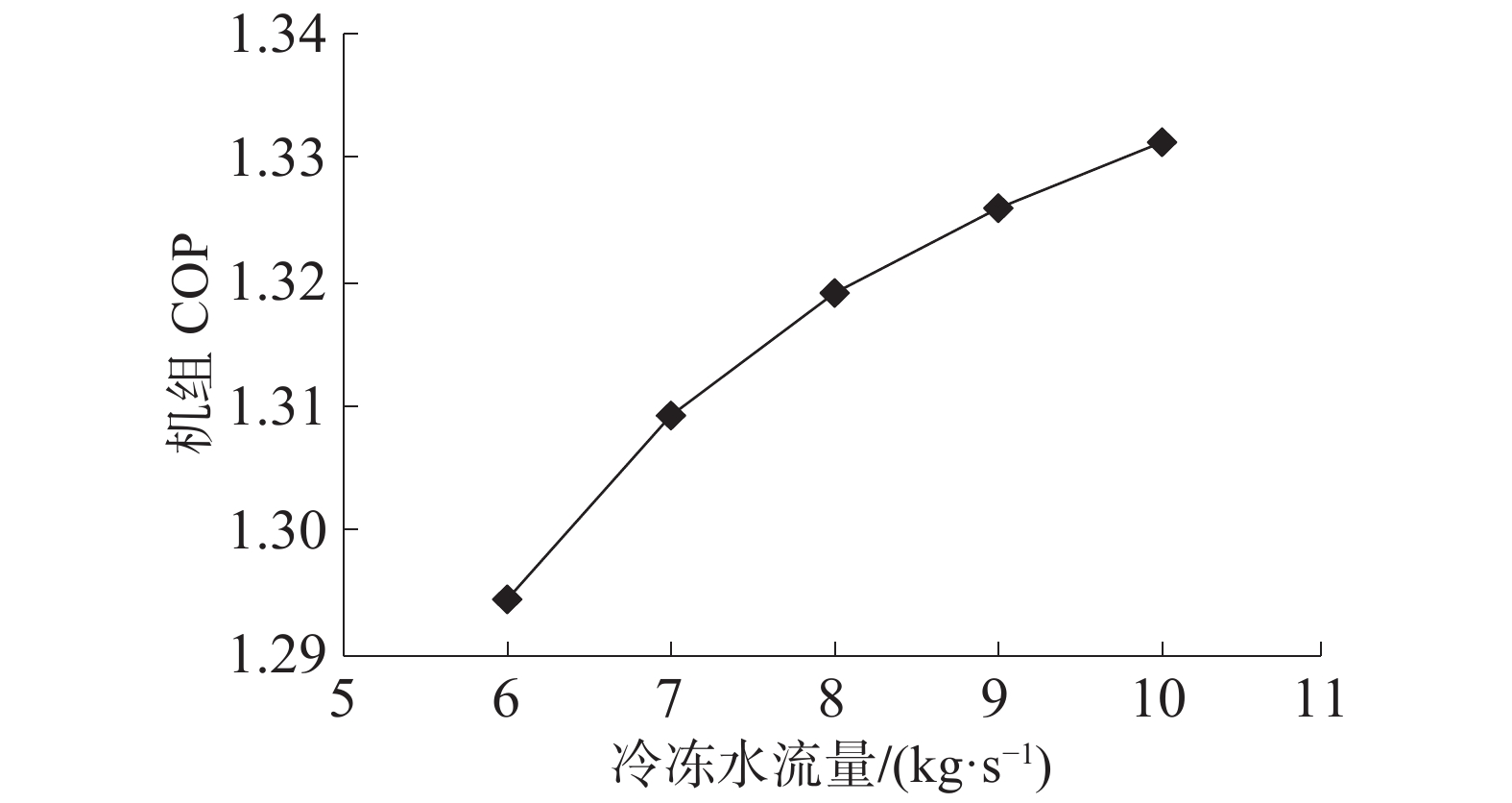Download: 图 6 冷冻水流量对机组COP的影响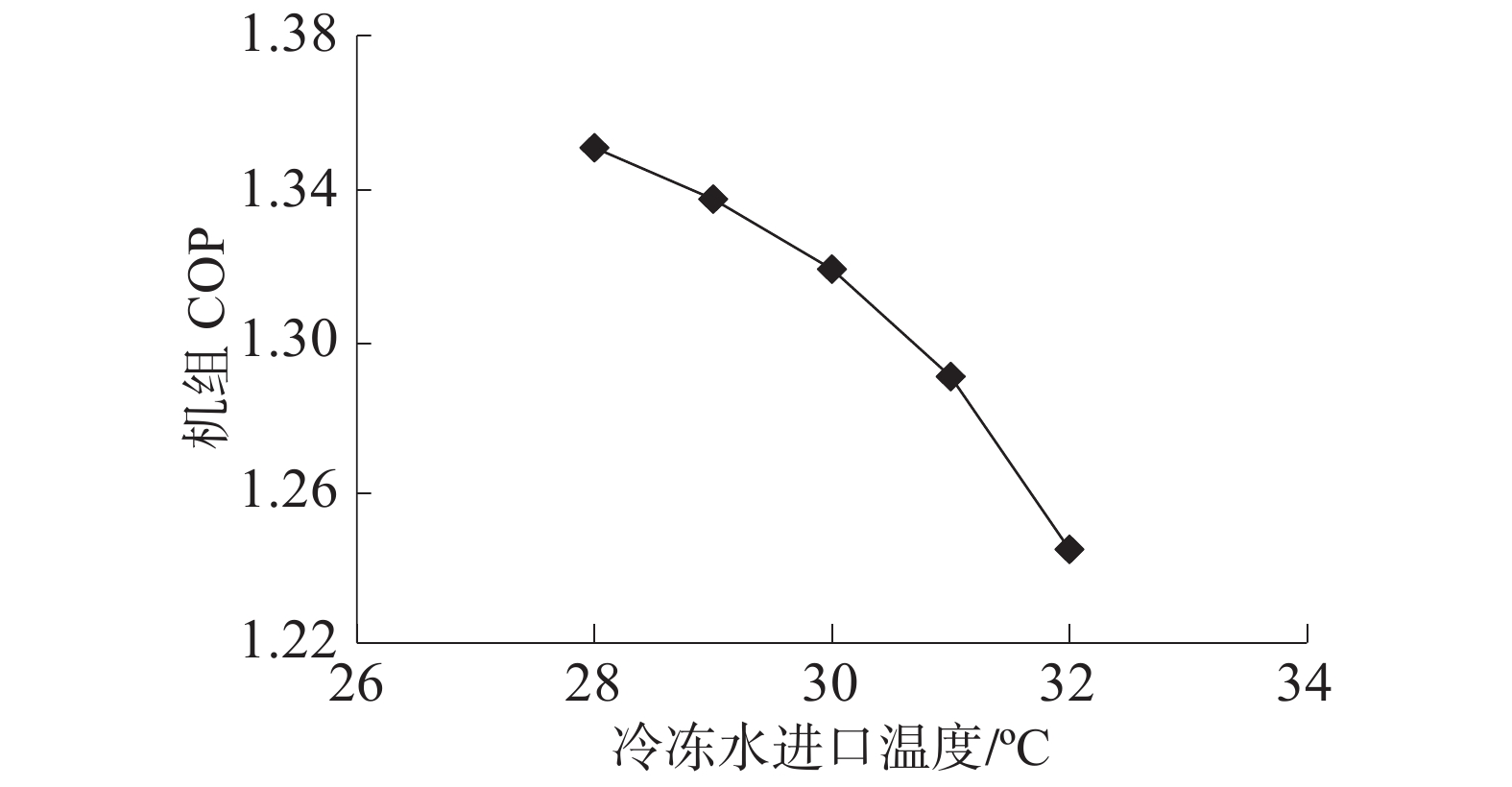Download: 图 7 冷却水进口温度对机组COP的影响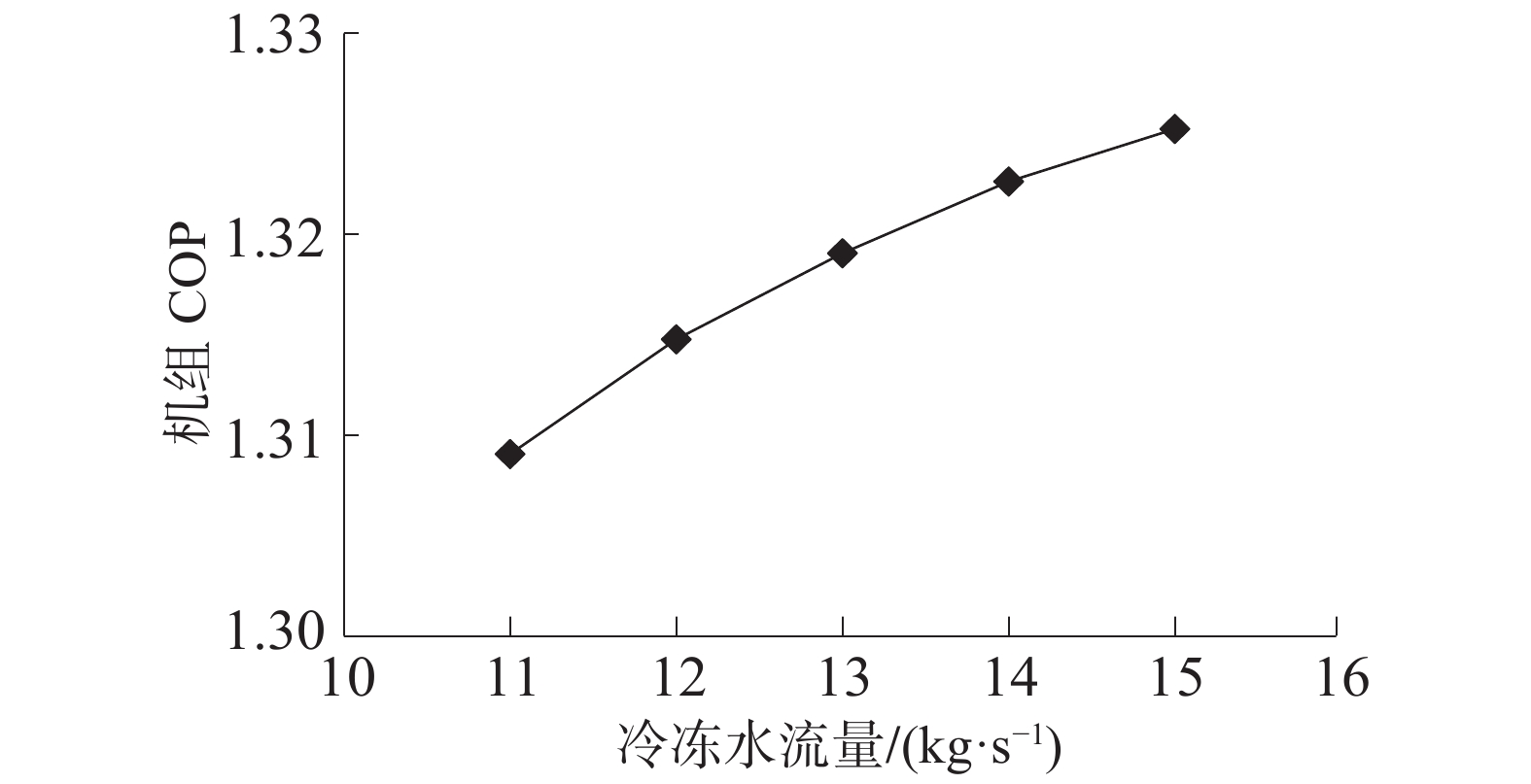Download: 图 8 冷却水流量对机组COP的影响

3 耗能计算分析

3.1 水下航行器运行工况表 1 水下航行器冷水机组运行工况
3.2 耗能计算分析

1) 设计计算表 2 冷水机组初步设计计算结果

2) 设计优化

F_hg：高压发生器换热面积，3个水平，41 、46、51 m2

F_lg：低压发生器换热面积，3个水平，51 、56、61 m2

F_e：蒸发器换热面积，3个水平，116 、121 、126 m2

F_c：冷凝器换热面积，3个水平，29 、34 、39 m2

F_hex：高温热交换器换热面积，3个水平，36 、41、46 m2

F_lex：低温热交换器换热面积，3个水平，16 、21 、26 m2

F_a：吸收器换热面积，3个水平，95 、100 、105 m2表 3 机组各设备换热面积优化设计正交表表 4 各组合计算结果表 5 冷水机组最终设计计算结果

3) 耗能分析

4 结论

1) 建立溴化锂吸收式冷水机组的数学模型，对溴化锂吸收式冷水机组进行实地测试。利用测试结果对模型进行验证，其中机组测试COP为1.07~1.38，模型计算COP最大相对误差为2.94%，可以认为该模型可用于预测溴化锂吸收式冷水机组性能。

2) 利用数学模型，对溴化锂吸收式冷水机组进行了性能分析，得到热源温度、冷却水进口温度以及冷冻水进口温度对机组COP的影响较大。

3) 通过某一水下航行器溴化锂冷水机组的设计优化计算，得到其设计COP为1.306 0；根据其运行条件，得到水下航行器冷水机组运行COP为1.29，乏汽耗量为0.380 kg/s，不足二回路蒸汽流量的0.6%，对于水下航行器的续航能力基本无影响。

  董鹏, 李志印, 简弃非. 大温差变风量送风技术在潜艇空调中的应用[J]. 中国舰船研究, 2012, 7(6): 90-97. (0)  林凡彩, 李建辉, 王希全. 潜艇的居住环境与个人防护[C]//船舶安全管理与防治污染管理学术研讨会. 哈尔滨, 中国, 2008:89-93. (0)  GJB 864-1990潜艇居住性规范[S]北京: 海装论证中心标准规范室.1990. (0)  钟民军, 陈金增, 吴钢. 某型潜艇空调能耗分析和节能研究[C]//全国船舶机电维修技术学术会议. 武汉, 中国, 2006:391-396. (0)  吴钢, 曾强洪, 陈金增, 等. 利用蓄冷空调提高潜艇水下续航力研究[J]. 船海工程, 2006, 35(6): 67-69. DOI:10.3963/j.issn.1671-7953.2006.06.021 (0)  李祥东, 汪荣顺. 水下密闭空间生存环境的综合改善技术−采用低温冷冻法改善潜艇舱室大气环境的空气净化系统[J]. 舰船科学技术, 2008, 30(6): 104-107. DOI:10.3404/j.issn.1672-7649.2008.06.021 (0)  马运义, 许建. 现代潜艇设计理论与技术[M]. 哈尔滨: 哈尔滨工程大学出版社, 2012. (0)  谢治祥, 陈兴华. 潜艇的空气调节[J]. 舰船科学技术, 2005, 27(1): 91-93. (0)  吴成强. 蒸气双效型溴化锂吸收式制冷机的应用[J]. 柳钢科技, 2003(3): 49. (0)  王大勇, 施耀华. 溴化锂吸收式制冷机的主要特点及操作注意事项[J]. 科技传播, 2010(18): 138–139. (0)  胡世华. 北方地区溴化锂吸收式制冷机组与电力冷水机组的选择[J]. 西安工程大学学报, 2000, 14(2): 191-194. DOI:10.3969/j.issn.1674-649X.2000.02.020 (0)  夏军宝, 李毅, 黎春梅, 等. 水下航行器冷水机组设计优化研究[J]. 制冷与空调, 2019, 19(12): 44-50. (0)  KAITA Y. Simulation results of triple-effect absorption cycles[J]. International journal of refrigeration, 2002, 25(7): 999-1007. DOI:10.1016/S0140-7007(01)00100-1 (0)  杨筱静. 蒸汽型双效溴化锂吸收式热泵机组性能及优化研究[D]. 天津: 天津大学, 2012. (0)  夏军宝. 槽式太阳能吸收式制冷及热水供暖系统地区应用研究[D]. 天津: 天津大学, 2018. (0)  徐勇. 槽式太阳能吸收式空调系统研究[D]. 北京: 华北电力大学, 2015. (0)  杨世铭, 陶文铨. 传热学(第3版) [M]. 北京: 高等教育出版社, 1998. (0)  COLEMAN T F, LI Y. On the convergence of interior-reflective Newton methods for nonlinear minimization subject to bounds[M]. Springer-Verlag New York, Inc. 1994. (0)  侍茂崇. 海洋与人类文明[M]. 哈尔滨: 哈尔滨工程大学出版社, 2008. (0)  王奎民. 主要海洋环境因素对水下航行器航行影响分析[J]. 智能系统学报, 2015, 10(2): 316-323. (0)  于俊崇. 船用核动力[M]. 上海: 上海交通大学出版社, 2016. (0)